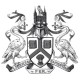# Mathematics Challenge

For a chance to win the first prize (£100 Amazon voucher), or one of our prizes for runners-up, submit your typed or neatly written solutions of the following problems to maths@lincoln.ac.uk or by post to Mathematics Challenge, School of Mathematics and Physics, University of Lincoln, Lincoln, LN6 7TS. Please include your full name, postal address and email, as well as the name and address of your school. The closing date is 5 January, 2016. The competition is open to all young pre-university people in UK aged 16–18 years. It is not open to current university students. See full Terms and Conditions.

1. Suppose that an underground tunnel of length 100 km is constructed strictly along a straight line between two points on the Earth’s surface. Assuming that the Earth is a perfect sphere of radius 6,371 km, estimate the maximum depth which the tunnel reaches below ground.

2. Find all solutions of the equation$4\cdot\big| |x|-2\big|= x+4$.

3. Prove that a number 2n , where n is a positive integer, cannot have four equal right-most digits.

4. In the following picture there are three squares of side length 1. Find the area of the triangle ABC.5. Prove that if a,b,c are odd integers, then the equation  ax2 + bx + c = 0 has no rational roots.

6. Every square of a 2015 × 2015 table contains either 1 or –1. It is known that the sum of the numbers in every 2 × 2  square is equal to zero. Prove that the sum of all numbers in the table cannot be greater than 2015.

Notes: Full solutions are required – not just answers – with complete proofs of any assertions you may make. A winning submission may not necessarily be based on all six problems – so you are encouraged to submit solutions even if you do only some of the problems.

1.Winners of the Mathematics Challenge 2015-16 – Maths & Physics News says: